Courses

# Test: Reaction Intermediate- 1

## 30 Questions MCQ Test Organic Chemistry | Test: Reaction Intermediate- 1

Description
This mock test of Test: Reaction Intermediate- 1 for Chemistry helps you for every Chemistry entrance exam. This contains 30 Multiple Choice Questions for Chemistry Test: Reaction Intermediate- 1 (mcq) to study with solutions a complete question bank. The solved questions answers in this Test: Reaction Intermediate- 1 quiz give you a good mix of easy questions and tough questions. Chemistry students definitely take this Test: Reaction Intermediate- 1 exercise for a better result in the exam. You can find other Test: Reaction Intermediate- 1 extra questions, long questions & short questions for Chemistry on EduRev as well by searching above.
QUESTION: 1

Solution:
QUESTION: 2

Solution:
QUESTION: 3

### The relative acidity of the indicated H in each of the following is: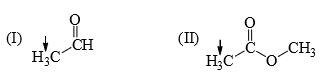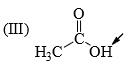Solution:
QUESTION: 4

The relative reactivity towards reaction with MeMgBr of the following is: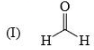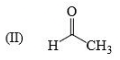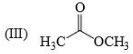Solution:
QUESTION: 5

The relative nucleophilicity in polar, protic, solvents of the following is: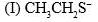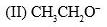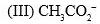Solution:

Explanation : In a polar protic solvent (CH3OH), nucleophilicity increases down a column of the periodic table. So S- is more nucleophilic than O-.

For two species with the same attacking atom, the more basic is the more nucleophilic, so CH3CH2O- is more nucleophilic than CH3CO2-

CO2- give identical str resonance hybrids more solvated, very less nucleophilic.

QUESTION: 6

The acidity of the protons H in each of the following is: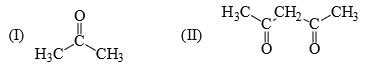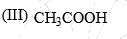Solution:
QUESTION: 7

The relative nucleophilicity in polar, protic, solvents of the following is:
(I) CH3OH                  (II) CH3SH                (III) CH3NH2

Solution:
QUESTION: 8

Reactivity of the following towards reaction with NaBH4 is: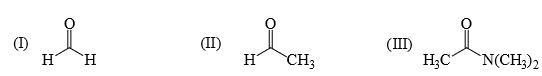Solution:
QUESTION: 9

Rate of reaction of CH3COCl/AlCl3 with each of the following is: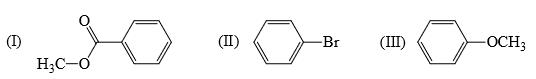Solution:
QUESTION: 10

Identify correct C—O bond length order: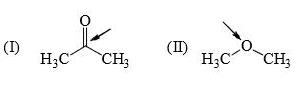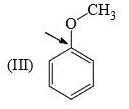Solution:

I is the shortest because of double bond.

III is next because of resonance.

II is last as there is no kind of bond strengthening.

Hence B is correct.

QUESTION: 11

The resonance energy of each of the following is: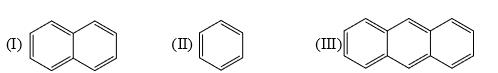Solution:
QUESTION: 12

Identify order of per ring resonance energies of each of the following: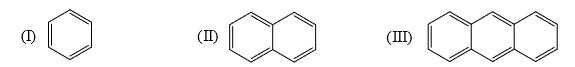Solution:
QUESTION: 13

The relative stability of the following carbocations is: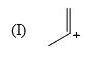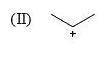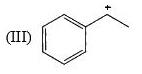Solution:

Explanation : The dispersal of the charge stabilizes the carbocation. More the number of alkyl groups, the greater the dispersal of positive charge and therefore, more the stability of carbocation,is an electron donating group, thus it will increases the stability of carbocation, hence the expected order is, (iii)>(ii)>(i).

QUESTION: 14

Which of the following systems are resonance contributions of the radical shown below: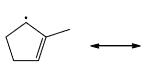Solution:
QUESTION: 15

Imidazole has a pKa = 7 with respect to its conjugate acid. Which N is protonated in this conjugate acid and why?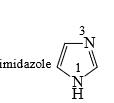Solution:
QUESTION: 16

Cyclopentadiene has a pKa = 15, whereas cyclopentane has a pKa > 50. This is because:

Solution:

Since after deprotonation cyclopentadiene becomes stable by aromaticity, So, it will definitely have a higher acidic strength than that of cyclopentane and consequently lower pKa value.

Further, no such stability factor has a role in the case of cyclopentane so it has lower acidic strength comparatively.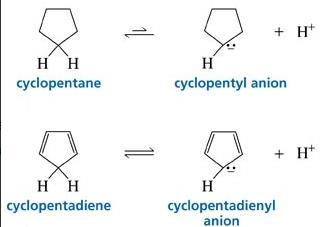QUESTION: 17

Which of the following is most likely to undergo a favourable hydride shift?

Solution:
QUESTION: 18

Arrange the following intermediate into decreasing order of stability.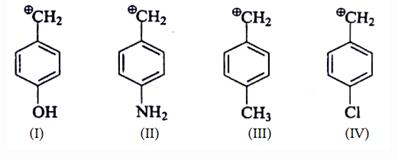Solution: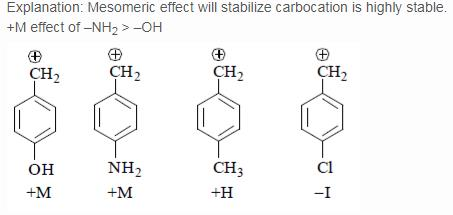QUESTION: 19

Which of the following forms most stable carbocation upon removal of OH–:

Solution:
QUESTION: 20

Which of the following carbonium ion is most stable?

Solution:
QUESTION: 21

Which of the following carbocations would not likely rearrange to a more stable carbocation?

Solution:
QUESTION: 22

Which carbocation is the most stabilized:

Solution:
QUESTION: 23

Which one of the following carbocation would you expect to rearrange?

Solution:

In option B and C, after carbocation rearrangement, it will remain 2°, therefore, rearrangement will not take place.
In option D, after carbocation rearrangement 3° will become 2° or 1°, therefore, rearrangement will not take place.
In option A, after rearrangement, carbocation will become 3° from 2°, therefore, this rearrangment will take place.

QUESTION: 24

Which carbocation is the most stable?

Solution:
QUESTION: 25

Arrange stability of the given carbocations in decreasing order: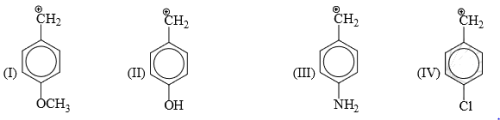Solution:
QUESTION: 26

In each of the following pairs of ions, which ion is more stable:

Solution:
QUESTION: 27

Consider the following statements: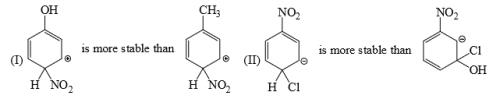Solution:
QUESTION: 28

Which is the most stable arenium carbocation:

Solution:
QUESTION: 29

Which of the following statements is correct: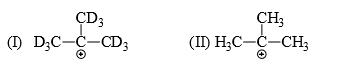Solution:
QUESTION: 30

List the following carbocations in order of decreasing stability (starting with the most stable):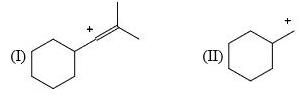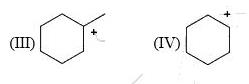Solution: